Arrange their stabilities of given gem- diols in decreasing order.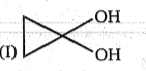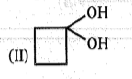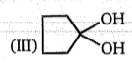(a) I > II > III

(b) III > II > I

(c) I > III > II

(d) III > I > II

Concept Questions :-

Alcohols: Preparation, Physical and Chemical Properties
High Yielding Test Series + Question Bank - NEET 2020

Difficulty Level: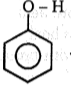$\stackrel{{\mathrm{HNO}}_{3}/{\mathrm{H}}_{2}{\mathrm{SO}}_{4}}{\to }$ (A)                  +               (B)

(more volatile)                     (less volatile)

Product (A) of the above reaction is: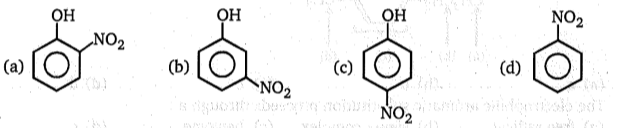Concept Questions :-

Phenols: Preparation, Properties, Acidic Nature of Phenol
High Yielding Test Series + Question Bank - NEET 2020

Difficulty Level:

What is the product obtained by heating the following allylic ether of phenol?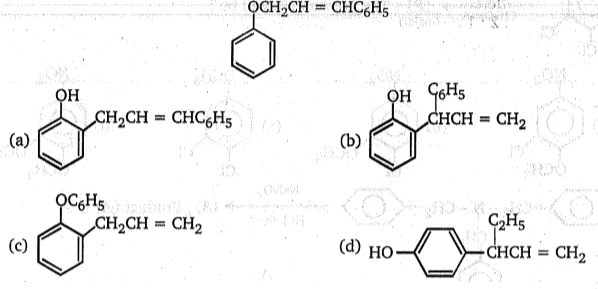Concept Questions :-

Phenols: Preparation, Properties, Acidic Nature of Phenol
High Yielding Test Series + Question Bank - NEET 2020

Difficulty Level: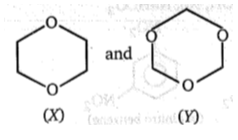; Compounds (X) and (Y) can be differentiated by:
(a) ${\mathrm{H}}_{3}{\mathrm{O}}^{\oplus }$, NaOI
(b) ${\mathrm{H}}_{3}{\mathrm{O}}^{\oplus }$, then Fehling test
(c) ${\mathrm{H}}_{3}{\mathrm{O}}^{\oplus }$, then Na
(d) Both (b) and (c)

Concept Questions :-

Ethers: Preparation, Physical & Chemical Properties, Uses
High Yielding Test Series + Question Bank - NEET 2020

Difficulty Level:

Which of the following compounds give positive Tollen's Test?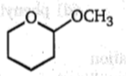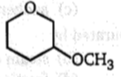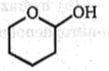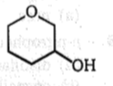(a)                           (b)                           (c)                            (d)

Concept Questions :-

Alcohols: Preparation, Physical and Chemical Properties
High Yielding Test Series + Question Bank - NEET 2020

Difficulty Level:

Test to differentiate between (CH3OH) and (Ph-OH) is/are:

(a) Litmus test

(b) FeCl3

(c) Br2/H2O

(d) All of these

Concept Questions :-

Alcohols: Preparation, Physical and Chemical Properties
High Yielding Test Series + Question Bank - NEET 2020

Difficulty Level:

In the reaction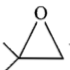+ CH3OH $\stackrel{{\mathrm{CH}}_{3}\mathrm{ONa}}{\to }$Product.

The product is:

(a)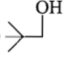(b)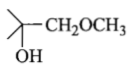(c)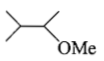(d)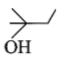Concept Questions :-

Ethers: Preparation, Physical & Chemical Properties, Uses
High Yielding Test Series + Question Bank - NEET 2020

Difficulty Level:

Methanol and ethanol can be distinguished by the following:

(a) by reaction with metallic sodium

(b) by reaction with caustic soda

(c) by heating with iodine and washing soda

(d) by heating with zinc and inorganic mineral acid

Concept Questions :-

Mechanism of Dehydration, Uses, Methanol and Ethanol
High Yielding Test Series + Question Bank - NEET 2020

Difficulty Level:

Which of the following methods cannot be used for the preparation of an ester?

(a) RCOOH+ R'OH+OH-
(b) RCOCl+R'OH+Pyridine
(c) RCOOH+R’OH+H+
(d) (RCO)2O+R'OH+Pyridine

Concept Questions :-

Alcohols: Preparation, Physical and Chemical Properties
High Yielding Test Series + Question Bank - NEET 2020

Difficulty Level:

Methylated spirit is:

(a) methanol containing some pyridine

(b) ethanol containing some methanol

(c) pure methanol

(d) 95% methanol

Concept Questions :-

Alcohols: Preparation, Physical and Chemical Properties